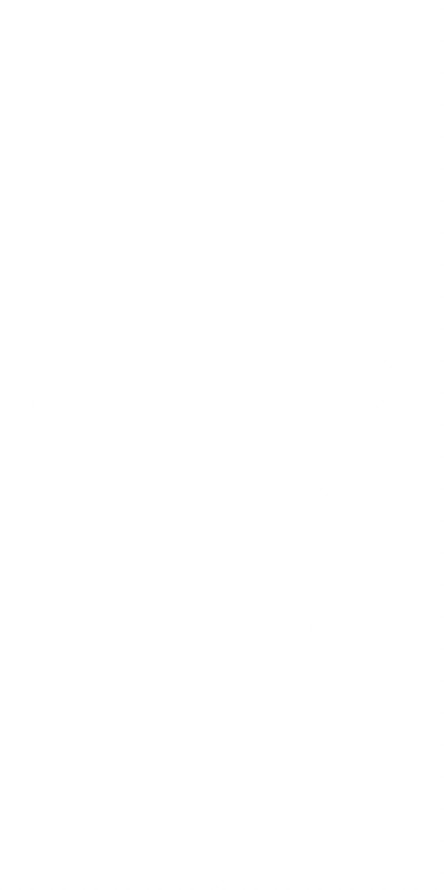# Calculus 03Quick Scroll turns off the high-resolution images to make scrolling smoother. Turn it on and off with the lightning button in the menu .
  Quick Scroll is onoff
College Level
Coming SoonVectors and 3D Space
Partial Derivatives
Multiple Integrals
Vector Calculus
Vectors and 3D Space
Partial Derivatives
Multiple Integrals
Vector Calculus
Vectors and 3D Space
Partial Derivatives
Multiple Integrals
Vector Calculus
Vectors and 3D Space
Partial Derivatives
Multiple Integrals
Vector Calculus
Vectors and 3D Space
Partial Derivatives
Multiple Integrals
Vector Calculus
Vectors and 3D Space
Partial Derivatives
Multiple Integrals
Vector Calculus

# Unit 1: Vectors and 3D Space

This unit is coming soon!

# Unit 2: Partial Derivatives

This unit is coming soon!

# Unit 3: Multiple Integrals

This unit is coming soon!

# Unit 4: Vector Calculus

This unit is coming soon!